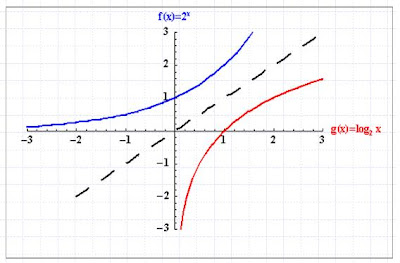## Wednesday, October 10, 2007

### Learning basic maths froom Om

 class 1 you learn numbers 0, 1, 2, 3, 4....9 class 3 you learn additions 1+1=2class 5 you learn multiplications means you learned shortcut for addition 4 + 4+ 4=3•4 class 8 you learn Exponents(power) means you learned shortcut for multiplication 2•2•2 =23 class 10 you learn Logarithm means you learned shortcut for exponents 10•10=102=log10(100)=2 and in class 12 you learn use of all this classicsClassic use of logHow long would it take a town of 2000 people to grow to 2500 people at a rate of 7% annually?Soln: use log with P=P0(1+r)?

Comments on "Learning basic maths froom Om"